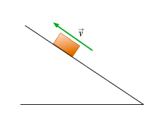# Problem: Now consider a block sliding up a rough slope after having been given a quick push as shown. Which of the following forces act on the block?a. force of pushb. kinetic frictionc. weightd. static frictione. normal forcef. the force of velocity

###### FREE Expert Solution

The block has been given a quick push and left to slide up.

This tells us that there is no force of push acting on the block.

81% (203 ratings)###### Problem DetailsNow consider a block sliding up a rough slope after having been given a quick push as shown. Which of the following forces act on the block?
a. force of push
b. kinetic friction
c. weight
d. static friction
e. normal force
f. the force of velocity

Frequently Asked Questions

What scientific concept do you need to know in order to solve this problem?

Our tutors have indicated that to solve this problem you will need to apply the Motion On Inclined Planes concept. If you need more Motion On Inclined Planes practice, you can also practice Motion On Inclined Planes practice problems.